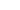Video player is disabled while the embed window is open.

Copy and paste the HTML code below:

Color:
Size: X

|

| |

Tour De Liozinec

The greatest 2012 bike race!

• + 8Niksancho96 (Apr 12, 2012 at 0:56)
VOD!
• + 6crowaan (Apr 12, 2012 at 11:04)
I think the XC rider won in the end. Hilarious video.
• + 4TihoDobrev (Apr 12, 2012 at 9:03)
VOD, VOD , VOD!!!
:bowdown:
• + 4ExiTeYeS (Apr 11, 2012 at 12:31)
Eto go sledvashtiq razbivasht klip! Dano i tozi pyt stane VOD!
• + 4nasrannn (Apr 12, 2012 at 10:03)
One funny and awesome video ! VOD !!!
• + 5AirBorneone101 (Apr 12, 2012 at 0:13)
VOD!!!
• + 4freeridejump (Apr 12, 2012 at 9:44)
VOD VOD !!!!!
• + 3ninjea (Apr 11, 2012 at 15:35)
siiick
• + 3lusi5001 (Apr 12, 2012 at 10:21)
VOD
• + 3Stanev (Apr 12, 2012 at 10:41)
Great video! VOD!
• + 2KempeleenPoika (Apr 13, 2012 at 2:11)
Bmx guys won definitely.
• + 2ddiidd (Apr 13, 2012 at 2:05)
VOD!
• + 1darlik95 (Apr 14, 2012 at 8:31)
What's the song at the end?
• + 2mtbvein (Sep 7, 2012 at 6:03)
i was expeting more dh
• + 2deathmetaldownhill (Apr 27, 2012 at 12:07)
VOD. Big lols!
• + 2mopper (Apr 14, 2012 at 10:54)
VOD!!!
• + 2darlik95 (Apr 14, 2012 at 8:34)
VOD!
• + 2Rystio (Apr 13, 2012 at 1:30)
VOD!!!
• + 2AlexanderBG (Apr 12, 2012 at 11:11)
VOD
• + 2Aikata (Apr 12, 2012 at 21:57)
Voood
• + 2hristoto (Apr 12, 2012 at 6:24)
VOD
• + 2VOLATGE (Apr 12, 2012 at 3:12)
VOD
• + 2saizswut (Apr 12, 2012 at 8:41)
vod
• + 2stefan-ram (Apr 12, 2012 at 6:59)
vod• + 2ilian1000 (Apr 12, 2012 at 13:09)
VOD
• + 2vvvaaal (Apr 12, 2012 at 13:39)
VOD
• + 1asenchbg (Apr 18, 2012 at 14:55)
tosho = vod
• + 1go6obg (Jul 15, 2012 at 4:41)
VOD!!!!!
• + 1nouser (Apr 18, 2012 at 14:54)
POD!!!
• + 1kasmer (Apr 13, 2012 at 5:30)
VOD
• + 1Ivo-Jordanov (Apr 17, 2012 at 5:51)
VOD!!!